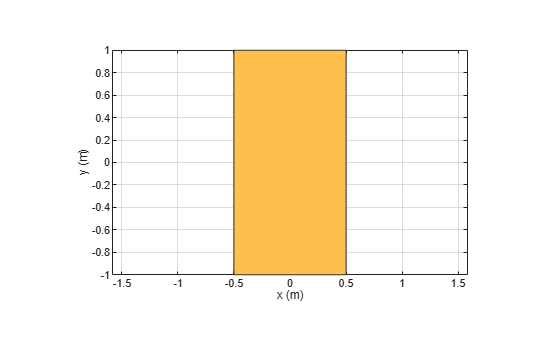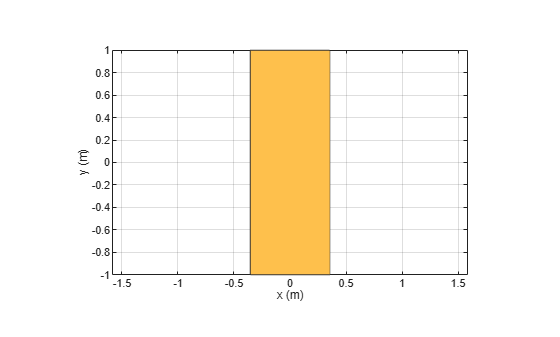# rotateY

Rotate shape about Y-axis and angle

## Syntax

``rotateY(shape,angle)``
``c = rotateY(shape,angle)``

## Description

example

````rotateY(shape,angle)` rotate shape about the Y-axis and angle.```
````c = rotateY(shape,angle)` rotate shape about the Y-axis and angle.```

## Examples

collapse all

Create a rectangle shape.

```r = antenna.Rectangle; show(r) axis equal```Rotate the rectangle at 45 degrees about the Y-axis.

`r1 = rotateY(r,45)`
```r1 = Rectangle with properties: Name: 'myrectangle' Center: [0 0] Length: 1 Width: 2 NumPoints: 2 ```
```show(r1) axis equal```## Input Arguments

collapse all

Shape created using custom elements and shape objects of Antenna Toolbox, specified as an object handle.

Example: `rotateY(rectangle)` where rectangle is created using `antenna.Rectangle` object.

Angle of rotation, specified as a scalar in degrees

Example: `rotateY(rectangle,45)` rotates the rectangle around Y-axis by 45 degrees.

Data Types: `double`